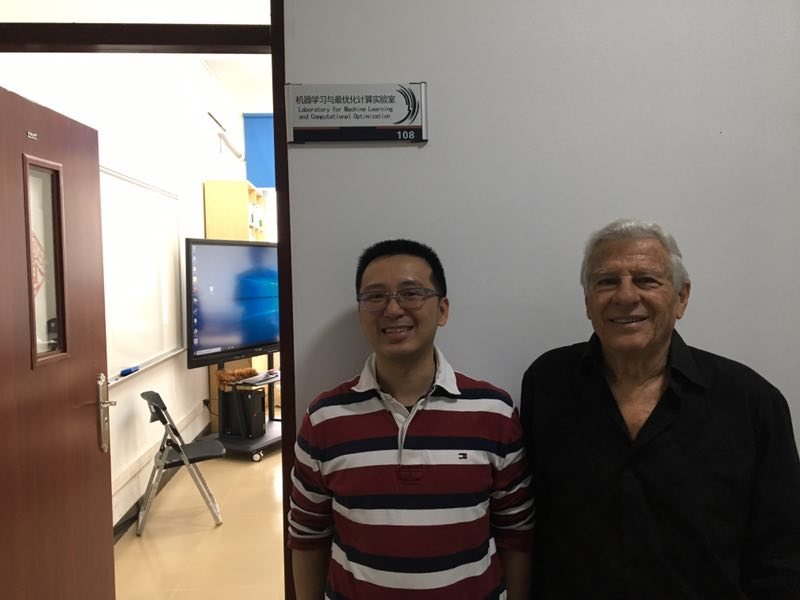Colloquiums and Conferences

# Interpolatory Pointwise Estimates for Monotone and Convex Piecewise-Polynomial Approximation

Time: 2019-02-24 20:58:47

Topic：Interpolatory Pointwise Estimates for Monotone and Convex Piecewise-Polynomial Approximation

Speaker：Dany Leviatan    Tel Aviv University in Israel

Time：2019-02-15 10:30--11:30

Location：Room 108 in South China Research Center for Applied Mathematics and Interdisciplinary Studies

Introduction：

Given a monotone or convex function f, we obtain pointwise estimates for its monotone,respectively, convex approximation by piecewise polynomials involving the second order modulus of smoothness of f^r. These estimates are interpolatory estimates, namely, the piecewise polynomials interpolate the function at the end-points of the interval. However,they are valid only for n > N(f,r). We also show thatsuch estimates are in general invalid with N independent of f. Note that the above estimate in the case r = 0 is achieved by piecewise linears interpolating f at the Chebyshev nodes. This was proved in the early 1980's by DeVore and Yu (for monotone approximation) and by Leviatan (for convex approximation).